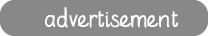Welcome to ictgames. Here you'll find free to play educational games aimed at children between 5 and 8 years old; written by a UK teacher with 20 years experience. The games are linked to the UK KS 1 and 2 curricula. Here are some help videos to get you started.
Teaching Tools
Printables
Topic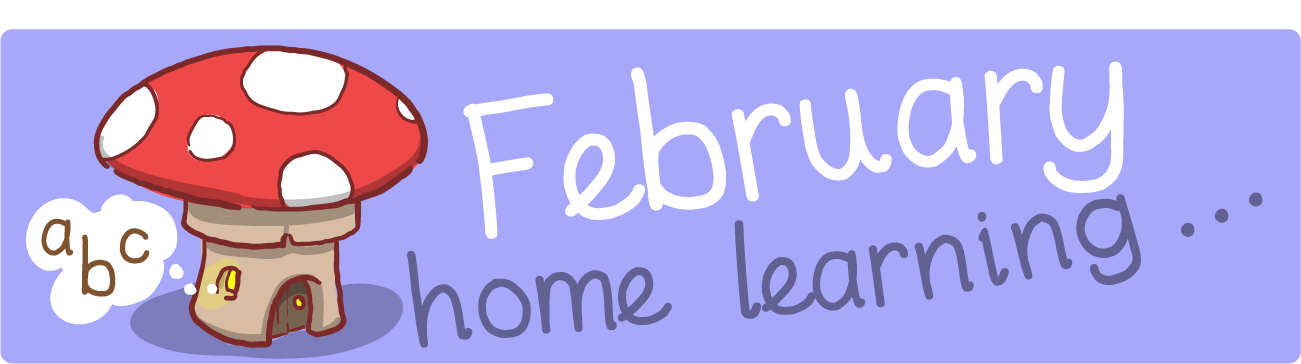Number - number and place valuePlace valuePlace ValuePlace Value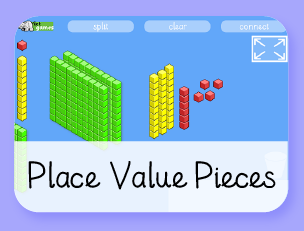Place ValuePlace Value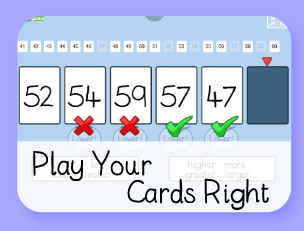Counting & OrderingCounting / Decimals / +10VariousCountingCountingCountingNumber SpellingOrdering NumbersOrdering numbersOrdering numbersOdds & Evens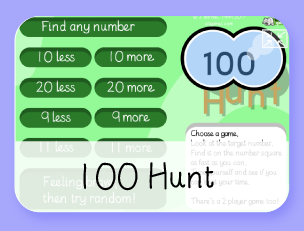+/- 10, 9, 11CountingCounting & SubtractionPlace Value & Decimals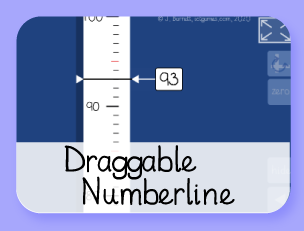CountingCounting & Subtraction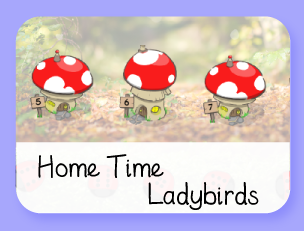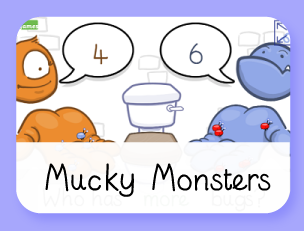Comparing NumbersNumber FactsNumber FactsSubtraction+/- 10, 9, 11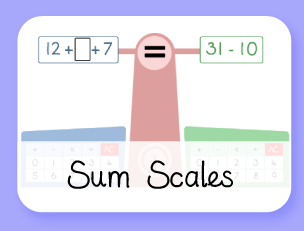Missing NumbersSubtraction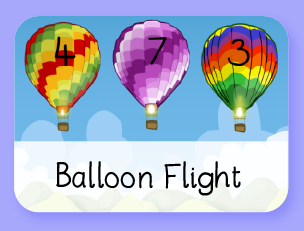Number Facts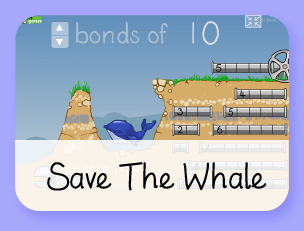Bonds of 10Number factsSubtraction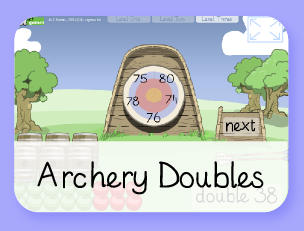DoublesBridging to 10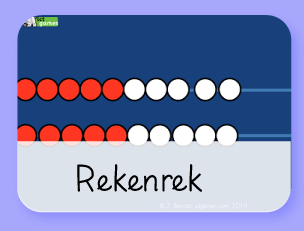+/-/x Facts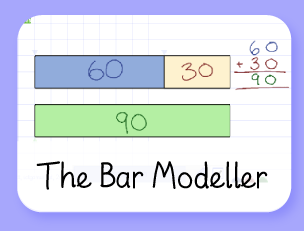Word Problems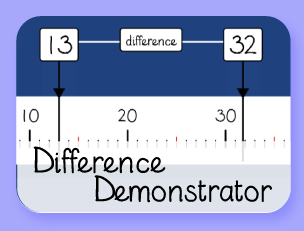Subtraction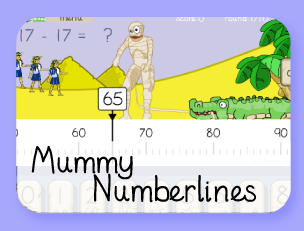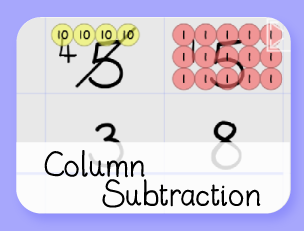Subtraction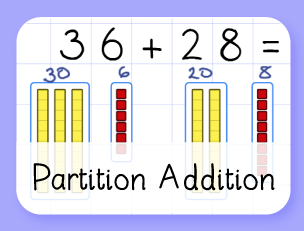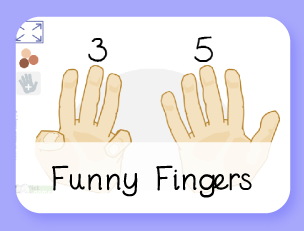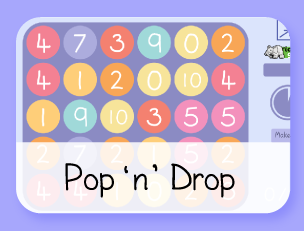Number - multiplication & divisionCountingTimes tablesMultiplication & Division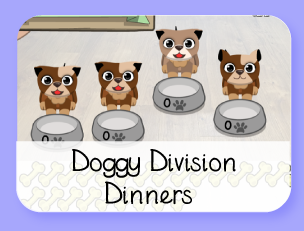DivisionNumber facts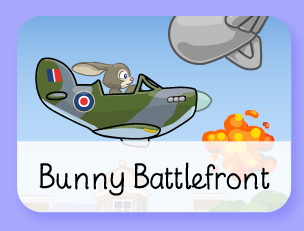Times Tables TestTimes Tables CountingMultiplication
Number - fractionsFractionsFractionsFractions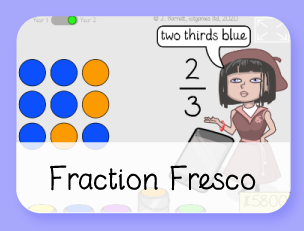FractionsFractions
Measurement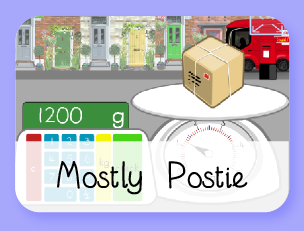Measure(g/kg)Measure(ml/l)Clock DemonstratorTimeMoneyMoneyTimerMoney
Geometry – properties of shapesShapeVariousThe Date Chart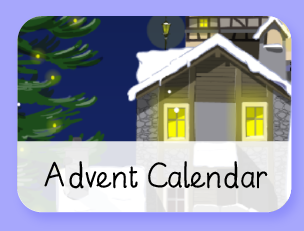Christmas